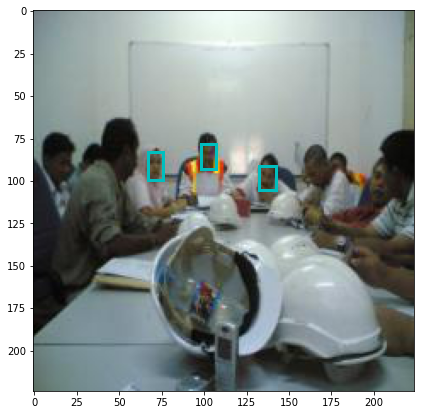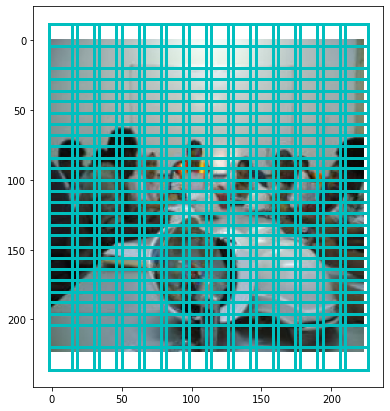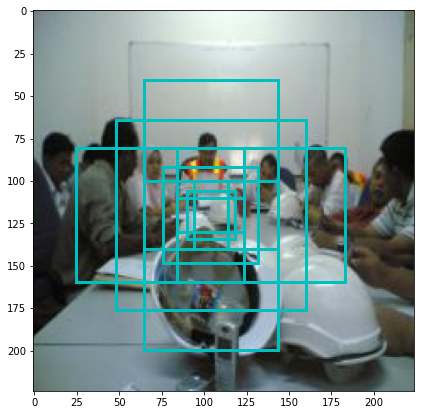# ECE 417 MP3: Face Detection using Faster RCNN¶

This MP is an implementation of the Faster RCNN object detector, to perform face detection using a small dataset extracted from the very large WIDER FACE dataset.

• The template package that you should download is here: http://courses.engr.illinois.edu/ece417/fa2020/ece417_20fall_mp3.zip.
• When you download and unzip that package, you will find a file called mp3_overview.ipynb. If you open that file using any ipython or Jupyter notebook, you will see a live version of the web page you are looking at right now.
• The only code that you will submit is the file mp3.py. The parts that you need to fill in are marked with NotImplementedErrors.
• As you edit mp3.py, you can see the results of your edits by running the corresponding code blocks in this file. Notice that each relevant code block starts with a importlib.reload(mp3), which will reload your most recent edited version, and run it.
• When everything on this page seems to run OK, you can run the unit tests by typing python run_tests.py or python run_tests.py -j in a console.
• When the unit tests all succeed on your own machine, you can try them on the autograder. Just upload the file mp3.py (if you upload other files, they will not be graded).

The rest of this file contains code for debugging mp3.py. If you are looking at this file on the web, you can browse the blocks below, to see what kind of material the MP will cover. If you are interacting with this file on your own machine, you can run the code yourself, to test your own version of mp3.py, and you can edit these code blocks as much as you want, in order to print out the variables, or any other debugging step that's useful to you.

In :
import numpy  as np
import matplotlib.pyplot as plt
%matplotlib inline
import matplotlib.figure
import importlib, os, sys, h5py


This block imports your mp3.py code:

In :
import mp3


## 0. Browsing the Data¶

First, we'll create a function that plots face rectangles on top of the image. Then we'll use it to look at some of the data.

In :
def show_image_with_rectangles(image,rects):
fig=plt.figure(figsize=(7,7))
plt.imshow(image)
for rect in rects:
x = rect+np.array([-rect/2,rect/2])
y = rect+np.array([-rect/2,rect/2])
plt.plot(x,y*np.ones(2),'c-',linewidth=3)
plt.plot(x,y*np.ones(2),'c-',linewidth=3)
plt.plot(x*np.ones(2),y,'c-',linewidth=3)
plt.plot(x*np.ones(2),y,'c-',linewidth=3)


It's good practice to use "importlib.reload" before you call anything from mp3.py. That way, every time you make changes to that file, your changes will be drawn in to this notebook page immediately.

This chunk of code loads in the mp3_dataset object. We'll load in the first datum, and find out what members it contains.

In :
importlib.reload(mp3)
mp3_dataset = mp3.MP3_Dataset('data')
datum=mp3_dataset
for k in datum.keys():
print('Object %s has shape %s'%(k,datum[k].shape))

Object image has shape (224, 224, 3)
Object features has shape (1, 512, 14, 14)
Object rects has shape (3, 10)
Object target has shape (9, 196, 5)


Next, let's plot the image, and overlay its reference rectangles on top:

In :
show_image_with_rectangles(datum['image'],datum['rects'])Next, let's plot the anchor rectangles. For reasons of efficiency, the anchors are stored in the opposite order: anchors[i,xy,a] is the i'th term in the rectangle corresponding to the a'tjh anchor (0<=a<=8) associated with the xy'th image position (0<=xy<=195). In order to make it easier to plot, let's create a transposed version.

In :
anchor_rects = np.transpose(mp3_dataset.anchors,[1,2,0])
[ mp3_dataset.anchors.shape, anchor_rects.shape ]

Out:
[(4, 196, 9), (196, 9, 4)]

In order to plot the smallest square at each anchor position, we just need to choose the zero'th anchor associated with each position.

In :
show_image_with_rectangles(datum['image'],anchor_rects[:,0,:])To better understand the anchors, let's show all of the anchors associated with a position somewhere out in the middle of the image -- say, position number x=7, y=7 (thus xy = 7x14+7-1=104).

In :
show_image_with_rectangles(datum['image'],anchor_rects[104,:,:])Finally: the classification targets and regression targets are encoded into the array called datum['target']. Specifically, the binary classification target for the a'th anchor at the i'th position is encoded as datum['target'][a,i,4], and if the classification target is 1, then the regression target is encoded as datum['target'][a,i,0:4].

Notice the min(np.log(2),regression_target) here. That keeps the computed rectangle from ever being more than twice as large as the anchor rectangle. I recommend that you use such a limit in your code, because sometimes the neural net outputs get very large.

In :
def target2rect(regression_target, anchor):
rect = np.zeros(4)
rect = regression_target*anchor+anchor
rect = regression_target*anchor+anchor
rect = np.exp(min(np.log(2),regression_target))*anchor
rect = np.exp(min(np.log(2),regression_target))*anchor
return(rect)


The Faster-RCNN coding scheme creates a target whenever a reference rectangle and an anchor rectangle have an IoU (intersection-over-union) greater than 0.7. For that reason, there are a lot more target rectangles than there were reference rectangles:

In :
target_rects = []
for a in range(9):
for i in range(196):
if datum['target'][a,i,4]==1:
rect = target2rect(datum['target'][a,i,0:4],anchor_rects[i,a,:])
target_rects.append(rect)
np.array(target_rects).shape

Out:
(416, 4)

If we plot the target rectangles, though, we should see that the number of distinct target rectangles is just the same as the number of reference rectangles. All of the extra targets are just duplicates of the same original references:

In :
show_image_with_rectangles(datum['image'],target_rects)